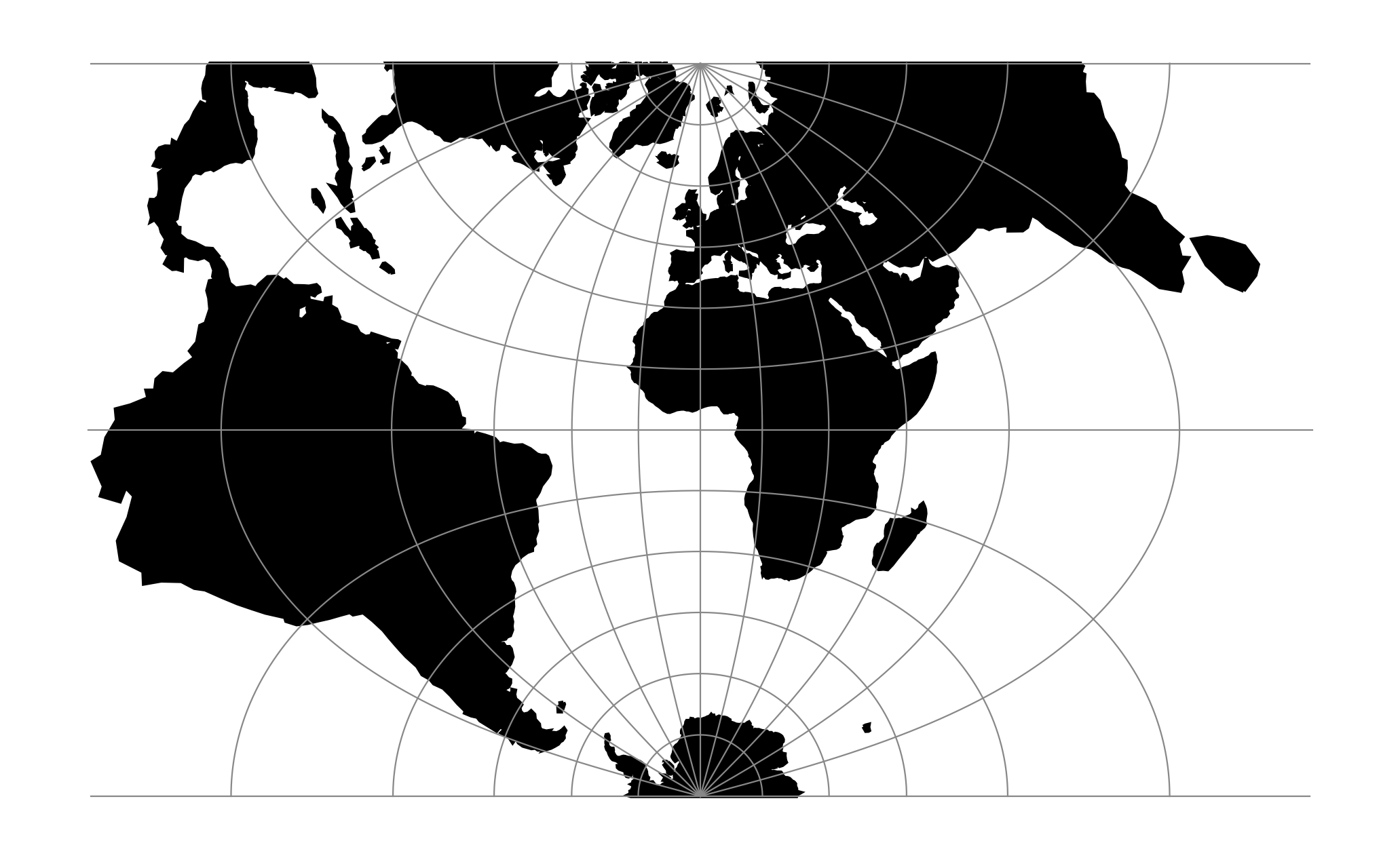# Transverse Mercator¶

The transverse Mercator projection in its various forms is the most widely used projected coordinate system for world topographical and offshore mapping.

 Classification Transverse and oblique cylindrical Available forms Forward and inverse, Spherical and Elliptical Defined area Global, but reasonably accurate only within 15 degrees of the central meridian Alias tmerc Domain 2D Input type Geodetic coordinates Output type Projected coordinatesproj-string: +proj=tmerc

## Usage¶

Prior to the development of the Universal Transverse Mercator coordinate system, several European nations demonstrated the utility of grid-based conformal maps by mapping their territory during the interwar period. Calculating the distance between two points on these maps could be performed more easily in the field (using the Pythagorean theorem) than was possible using the trigonometric formulas required under the graticule-based system of latitude and longitude. In the post-war years, these concepts were extended into the Universal Transverse Mercator/Universal Polar Stereographic (UTM/UPS) coordinate system, which is a global (or universal) system of grid-based maps.

The following table gives special cases of the Transverse Mercator projection.

Projection Name Areas Central meridian Zone width Scale Factor
Transverse Mercator World wide Various less than 6° Various
Transverse Mercator south oriented Southern Africa 2° intervals E of 11°E 1.000
UTM North hemisphere World wide equator to 84°N 6° intervals E & W of 3° E & W Always 6° 0.9996
UTM South hemisphere World wide north of 80°S to equator 6° intervals E & W of 3° E & W Always 6° 0.9996
Gauss-Kruger Former USSR, Yugoslavia, Germany, S. America, China Various, according to area Usually less than 6°, often less than 4° 1.0000
Gauss Boaga Italy Various, according to area 0.9996

Example using Gauss-Kruger on Germany area (aka EPSG:31467)

$echo 9 51 | proj +proj=tmerc +lat_0=0 +lon_0=9 +k_0=1 +x_0=3500000 +y_0=0 +ellps=bessel +datum=potsdam +units=m +no_defs 3500000.00 5651505.56  Example using Gauss Boaga on Italy area (EPSG:3004) $ echo 15 42 | proj +proj=tmerc +lat_0=0 +lon_0=15 +k_0=0.9996 +x_0=2520000 +y_0=0 +ellps=intl +units=m +no_defs
2520000.00  4649858.60


## Parameters¶

Note

All parameters for the projection are optional.

+approx

New in version 6.0.0.

Use the algorithm described in section “Elliptical Form” below. It is faster than the default algorithm, but also diverges faster as the distance from the central meridian increases.

+lon_0=<value>

Longitude of projection center.

Defaults to 0.0.

+lat_0=<value>

Latitude of projection center.

Defaults to 0.0.

+ellps=<value>

See proj -le for a list of available ellipsoids.

Defaults to “GRS80”.

+R=<value>

Radius of the sphere given in meters. If used in conjunction with +ellps +R takes precedence.

+k_0=<value>

Scale factor. Determines scale factor used in the projection.

Defaults to 1.0.

+x_0=<value>

False easting.

Defaults to 0.0.

+y_0=<value>

False northing.

Defaults to 0.0.

## Mathematical definition¶

The formulas describing the Transverse Mercator below are quoted from Evenden’s [Evenden2005].

$$\phi_0$$ is the latitude of origin that match the center of the map. It can be set with +lat_0.

$$k_0$$ is the scale factor at the natural origin (on the central meridian). It can be set with +k_0.

$$M(\phi)$$ is the meridional distance.

### Spherical form¶

#### Forward projection¶

$B = \cos \phi \sin \lambda$
$x = \frac{k_0}{2} \ln(\frac{1+B}{1-B})$
$y = k_0 ( \arctan(\frac{\tan(\phi)}{\cos \lambda}) - \phi_0)$

#### Inverse projection¶

$D = \frac{y}{k_0} + \phi_0$
$x' = \frac{x}{k_0}$
$\phi = \arcsin(\frac{\sin D}{\cosh x'})$
$\lambda = \arctan(\frac{\sinh x'}{\cos D})$

### Elliptical form¶

The formulas below describe the algorithm used when giving the +approx option. They are originally from [Snyder1987], but here quoted from [Evenden1995]. The default algorithm is given by Poder and Engsager in [Poder1998]

#### Forward projection¶

$N = \frac{k_0}{(1 - e^2 \sin^2\phi)^{1/2}}$
$R = \frac{k_0(1-e^2)}{(1-e^2 \sin^2\phi)^{3/2}}$
$t = \tan(\phi)$
$\eta = \frac{e^2}{1-e^2}cos^2\phi$
$\begin{split}x &= k_0 \lambda \cos \phi \\ &+ \frac{k_0 \lambda^3 \cos^3\phi}{3!}(1-t^2+\eta^2) \\ &+ \frac{k_0 \lambda^5 \cos^5\phi}{5!}(5-18t^2+t^4+14\eta^2-58t^2\eta^2) \\ &+\frac{k_0 \lambda^7 \cos^7\phi}{7!}(61-479t^2+179t^4-t^6)\end{split}$
$\begin{split}y &= M(\phi) \\ &+ \frac{k_0 \lambda^2 \sin(\phi) \cos \phi}{2!} \\ &+ \frac{k_0 \lambda^4 \sin(\phi) \cos^3\phi}{4!}(5-t^2+9\eta^2+4\eta^4) \\ &+ \frac{k_0 \lambda^6 \sin(\phi) \cos^5\phi}{6!}(61-58t^2+t^4+270\eta^2-330t^2\eta^2) \\ &+ \frac{k_0 \lambda^8 \sin(\phi) \cos^7\phi}{8!}(1385-3111t^2+543t^4-t^6)\end{split}$

#### Inverse projection¶

$\phi_1 = M^-1(y)$
$N_1 = \frac{k_0}{1 - e^2 \sin^2\phi_1)^{1/2}}$
$R_1 = \frac{k_0(1-e^2)}{(1-e^2 \sin^2\phi_1)^{3/2}}$
$t_1 = \tan(\phi_1)$
$\eta_1 = \frac{e^2}{1-e^2}cos^2\phi_1$
$\begin{split}\phi &= \phi_1 \\ &- \frac{t_1 x^2}{2! R_1 N_1} \\ &+ \frac{t_1 x^4}{4! R_1 N_1^3}(5+3t_1^2+\eta_1^2-4\eta_1^4-9\eta_1^2t_1^2) \\ &- \frac{t_1 x^6}{6! R_1 N_1^5}(61+90t_1^2+46\eta_1^2+45t_1^4-252t_1^2\eta_1^2) \\ &+ \frac{t_1 x^8}{8! R_1 N_1^7}(1385+3633t_1^2+4095t_1^4+1575t_1^6)\end{split}$
$\begin{split}\lambda &= \frac{x}{\cos \phi N_1} \\ &- \frac{x^3}{3! \cos \phi N_1^3}(1+2t_1^2+\eta_1^2) \\ &+ \frac{x^5}{5! \cos \phi N_1^5}(5+6\eta_1^2+28t_1^2-3\eta_1^2+8t_1^2\eta_1^2) \\ &- \frac{x^7}{7! \cos \phi N_1^7}(61+662t_1^2+1320t_1^4+720t_1^6)\end{split}$